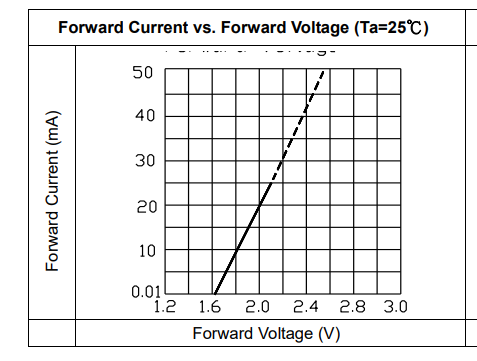# Calculate Load Resistor for LED Diode Circuit

There is a saying, the starting point of any software programming is to be able to print “Hello World” to a console. Similarly the simplest embedded programming task is to have MCU flash one LED using its GPIO pin.

To extend that, the simplest circuit design problem is to size that resistor value `R` in the following LED driver circuit.

## 1. I-V curve

In the circuit diagram above, `Vs` is the voltage source which is usually pre-determined as +9V, +5V or +3.3V. LED diode is chosen from one of many colors, thus having different I-V characteristics. The design goal is to decide what `R` value to use, to light up the LED with a certain current draw. In other words, to have LED bright enough without burning too much power. (We simplify the design problem a little bit, PWM isn’t considered here.)

If we split the single circuit loop into two halves. Looking into the right half, we see the single component: LED diode.

LED diode I-V curve, depending on its color, can be found in a bunch of diagrams (http://lednique.com/current-voltage-relationships/iv-curves/)

For example, a specific green LED I-V curve from the datasheet (https://datasheet.lcsc.com/lcsc/1809211715_Everlight-Elec-333-2SYGD-S530-E2-L_C99697.pdf)Green LED I-V curve: 20mA with 2.0V VF

The forward voltage VF and current IF (20mA) can also come from a table in the datasheet (https://cdn-shop.adafruit.com/datasheets/FLR-50T04-HW7+(2012.02.22).pdf)

You may notice that even the same color (green) LED, its VF voltage can be different from different manufacturers. Let’s pick one I-V curve as shown in “graph A”.

Now if we look into the left-half of the circuit, it consists of a voltage source in series with a resistor.

Its output characteristics I-V curve can be determined with two points on I-V axis as follows:

• if zero output current, no voltage drops on `R`, so output voltage equals the voltage source: `Vout = Vs` (i.e. i = 0, v = Vs)
• if the output is shorted, `Vout = 0`, so the loop current `I = Vs/R`. (i.e. v=0, i = Vs / R)

The resulting “graph B” is shown below.

## 2. Combine two I-V curves

So the trick is to merge these two halves of the circuits, thus two I-V curves (graph A and B) together, since`Vout = VLED`. In other words, where these two curves intersect, the resulting voltage and current satisfy both halves of the circuit.

In this case, a green LED already gives one data point from its datasheet: 20mA @ 2.3V, and it has to intersect with resistor I-V curve due to our design requirement. The rest of the voltage Vs — 2.3V is exactly the voltage drop on the resistor. Now it’s very easy to calculate the resistor value, assuming Vs = 3.3V

R = Vr / 20mA = (Vs — 2.3)/20mA = 1V/20mA = 50-Ohm

The advantage of this graph method is that you can change other parameters in the circuit and visualize the resulting changes on the graph directly.

For example, the following graph (blue trace) shows how as voltage source `Vs` increase, it drives up the LED current.

Another example, say you’d like to save power by reducing LED current to 15mA. You can certainly increase the resistor R.

But to increase by how much? This time slide rule comes to help.

We know that as the LED current drops, the cross point shifts left of 2.3V, which means Vr is more than 1V. Let’s estimate Vr = 1.2V.

On a slide rule, put Vr on C scale, and the LED current 15mA on D scale, the resulting C scale reads (from D=10) 80-Ohm on the right. (see my earlier post about placing the decimal point correctly; or just estimate it based on 50-Ohm result earlier)

The closest E24 resistor has value 82-Ohm. So we can slide C a little to the left, where 1.2V reads 14.6mA, which is close enough to 15mA.

## 3. Summary

Such graph method is also used for gain calculation of single stage transistor amplifier circuit.

This graph method used to be popular in analog circuit design, where linear circuitry (like the left hand side) meets non-linear (like the right hand side) component. Finding roots of non-linear equation numerically is equivalent to intersecting lines in this graph method.

Slide rule with voltage on C scale and current on D scale helps to read out resistor value directly. It’s useful for circuit design where a whole bunch of parameters can be “tried” all at once.

--

--